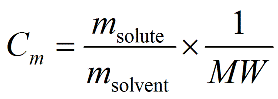PhysiologyWeb  Home  |  FAQ  |  About  |  Contact  |   - Sign InTweetMolal Solution Concentration Calculator
Meant to be used in both the teaching and research laboratory, this calculator (see below) can be utilized to perform a number of different calculations for preparing molal solutions when starting with the solid material.
If you wish to perform dilution calculations for solutions with molality concentration units, use our Molality Dilution Calculator.
If you wish to perform dilution factor or fold dilution calculations for solutions with molality concentration units, use our Dilution Factor Calculator - Molality.
Molal solution concentration equation• Cm is the molal concentration expressed in moles of solute dissolved per kilogram of solvent (mol/kg) (Molal or m). This is also referred to as molality, which is defined as the number of moles of solute dissolved per kilogram of solvent (mol/kg = m). A 1.00 m solution is one in which exactly 1.00 mole of solute is dissolved in exactly 1.00 kg of solvent. Using SI prefixes, the concentration may also be expressed in different fractions of the molal concentration such as mmol/kg (mm), μmol/kg (μm), nmol/kg (nm), pmol/kg (pm), etc. Note that molality is not the same as molarity, which is a common concentration unit that is defined as moles of solute dissolved in a defined total volume of solution (total solution volume refers to the final solution volume after the solute has been added to the solvent).
• msolute is the mass (i.e., weight) of solute in grams (g) that must be dissolved in mass msolvent of solvent in kilograms (kg) to make the desired molal concentration (Cm).
• msolvent is mass of solvent in kilograms (kg) in which the indicated mass of solute (msolute) in grams (g) must be dissolved to make the desired molal concentration (Cm).
• MW is the molecular weight in g/mol. Molecular weight is also referred to as formula weight and, in fact, many scientists prefer to use the latter. The molecular weight can be obtained from the molecular formula, data tables, or the label on the bottle containing the chemical of interest.
Molal solution concentration calculator
Each calculator cell shown below corresponds to a term in the formula presented above. Enter appropriate values in all cells except the one you wish to calculate. Therefore, at least three cells must have values, and no more than one cell may be blank. The value of the blank cell will be calculated based on the other values entered. After a calculation is performed, the calculated cell will be highlighted and subsequent calculations will calculate the value of the highlighted cell (with no requirement to have a blank cell). However, a blank cell has priority over a highlighted cell.
For convenience, this worksheet allows you to select different mass and concentration units, and the necessary conversions are carried out for you to obtain the value of the blank cell in the desired unit. Note that the unit of molecular weight must be g/mol.
 Molecular weight g/mol Mass of solute g pg ng μg mg g kg Mass of solvent kg pg ng μg mg g kg Concentration m fm pm nm μm mm m